# Statistics for SSC-CGL Tier-II (Paper-III) – Part-7

By | November 17, 2016

## FREQUENCY DISTRIBUTION

Data in a frequency array is ungrouped data. To group the data setting up of a 'frequency distribution' is required. A frequency distribution classifies the data into groups. It is simply a table in which the data are grouped into classes and the number of cases which fall in each class are recorded.  It shows   the frequency of occurrence of different values of a single Phenomenon.

### A frequency distribution is constructed for three main reasons:

1. To facilitate  the analysis of data.

2. To estimate  frequencies  of the unknown population distribution  from the distribution of sample data.

3. To facilitate the computation of various statistical measures.

### Raw data:

The statistical data collected are generally raw data or ungrouped data.  Let us consider the daily wages (in Rs ) of 30 labourers in a factory.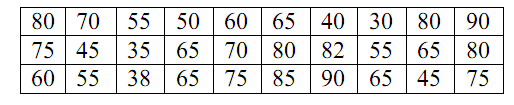The above figures are   nothing but raw or ungrouped data and they are recorded as they occur without any pre consideration. This representation of data does not furnish any useful information and is rather confusing to mind.  A better way to express the figures in an ascending or descending order of magnitude and is commonly known as array.  But this does not reduce the bulk of the data.  The above data when formed into an array is in the following form: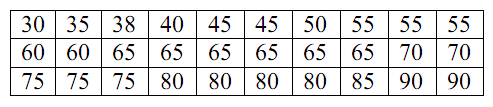The array helps us to see at once the maximum and minimum values.  It also gives a rough idea of the distribution of the items over the range . When we have a large number of items, the formation of an array is very difficult, tedious and cumbersome. The  Condensation should be directed for better understanding and may be done in two ways, depending on the nature of the data.

### a)  Discrete (or) Ungrouped frequency distribution:

In this form of distribution, the frequency refers to discrete value.   Here the data are presented in a way that exact measurement of units are clearly indicated. There are definite difference between the variables of different groups of items.  Each class is distinct and separate from the other class.  Non-continuity from one class to another class exist. Data as such facts like the number of rooms in a house, the number of companies registered in a country, the number of children in a family, etc.

The process of preparing this type of distribution is very simple.  We have just to count the number of times a particular value is repeated, which is called the frequency of that class.  In order to facilitate counting prepare a column of tallies.

In another column, place all possible values of variable from the lowest to the highest.  Then put a bar (Vertical line) opposite the particular value to which it relates.

To facilitate counting, blocks of five bars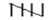are prepared and some space is left in between each block.  We finally count the number of bars and get frequency.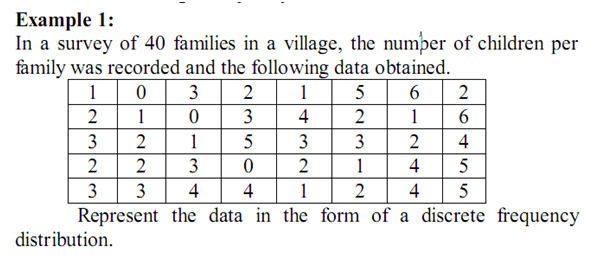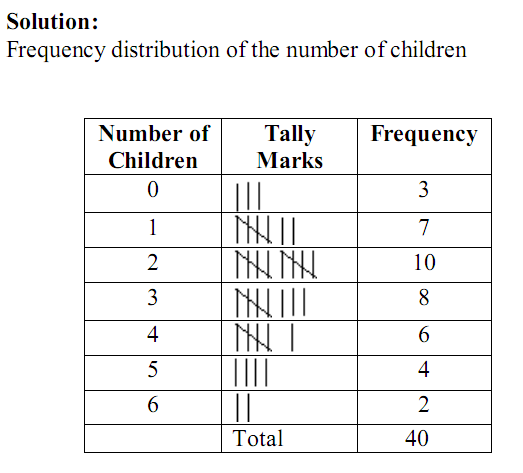b) Continuous frequency distribution:

In this form of distribution refers to groups of values.  This becomes necessary in the case of some variables which can take any fractional value and in which case an exact measurement is not possible.  Hence a discrete variable can be presented in the form of a continuous frequency distribution.

Wage distribution of 100 employees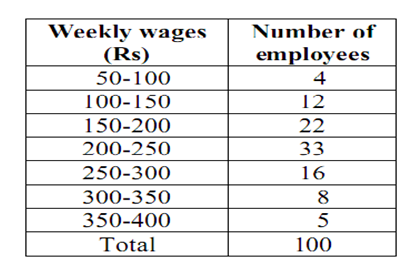## Nature of class

The following are some basic technical terms when a continuous frequency distribution is formed or data are classified according to class intervals.

### a) Class limits:

The class limits are the lowest and the highest values that can be included in the class.  For example, take the class  30-40. The lowest value of the class is 30 and highest class is 40.  The two boundaries of class are known as the lower limits and the upper limit of the class.  The lower limit of a class is the value below which there can be no item in the class.  The upper limit of a class is the value above which there can be no item to that class. Of the class 60-79, 60 is the lower limit and 79 is the upper limit, i.e. in the case there can be no value which is less than 60 or more than 79.  The way in which class limits are stated depends upon the nature of the data.  In statistical calculations, lower class limit is denoted by L and upper class limit by U.

### b) Class Interval (i):

The class interval may be defined as the size of each grouping of data.  For example, 50-75, 75-100, 100-125… are class intervals.  Each grouping begins with the lower limit of a class interval and ends at the lower limit of the next succeeding class interval. It is also called the class width.

### c) Mid-value or mid-point (M.V.):

The central point of a class interval is called  the mid value or mid-point.  It is found out by adding the upper and lower limits of a class and dividing the sum by 2.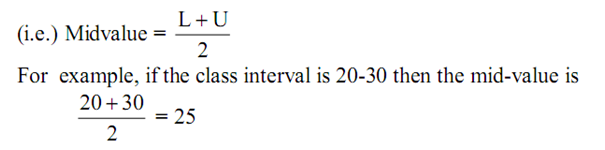### d) Class Frequency:

Number of observations falling within a particular class interval is called frequency of that class.

Let us consider the frequency distribution of weights if persons working in a company.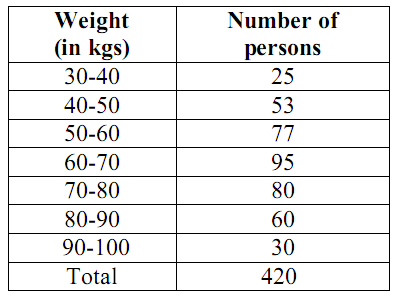In the above example, the class frequency are 25,53,77,95,80,60,30.  The total  frequency is equal to 420.  The total frequency indicate the total number of observations considered in a frequency distribution.